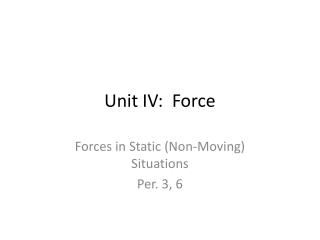DownloadDownload PresentationUnit IV: Force

# Unit IV: Force

Télécharger la présentation## Unit IV: Force

- - - - - - - - - - - - - - - - - - - - - - - - - - - E N D - - - - - - - - - - - - - - - - - - - - - - - - - - -
##### Presentation Transcript

1. Unit IV: Force Forces in Static (Non-Moving) Situations Per. 3, 6

2. Force: What is it? • Force is an interaction between two objects • Force is an interaction that can have an effect on the motion of an object • Has units of Newtons (N)

3. Consider the Rock below:

4. Notes on: Friction Force • Static Friction, Ffs , is a friction force exerted by a surface that prevents an object from moving. • Friction always OPPOSES motion • Friction never causes an object to move, it only resists motion • Friction is always directed parallel to the surface.

5. Notes on: Normal Force • Normal Force, FN , is the force exerted perpendicularly by a surface. • Normal Force is a reaction force; • It arises from the atoms or molecules in the surface pushing back, whenever they are pushed upon. • “Normal” is Math-speak for “Perpendicular” • Normal Force is always perpendicular to the surface.

6. Notes on: Tension Force • Force exerted along the length of an object, most commonly a rope, chain or string • Tension can be exerted by a rigid object (example, the handle of a wagon) • Tension is exerted along the length of the rope, string or chain. • Tension can be redirected by a pulley, it always goes the way the rope is going • For purposes of this class, Tension is constant within a rope, string or chain.

7. Drawing Force Diagrams • Identify a system (draw dotted line around the object that will be your system) • Identify every force acting on the system • Draw a Force vector arrow for every force • Vector goes in direction that force acts on the object • Magnitude represents relative strength • Label Force, FN , Fg, Ffs, etc. • Identify using “on-by”: FN onapplebyfloor

8. Half of Newton’s First Law • If an object is at rest, the Forces acting on that object are balanced • How can we judge whether forces are balanced?

9. Remember: • Friction Force parallel to surface, opposes motion • Normal Force perpendicular to the surface that is exerting the force • Tension Force along the length of the rope or string.